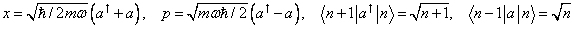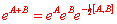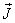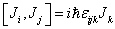# Physics 751: Midterm II Study Guide 2008

The best strategy is to read through my web notes from General Uncertainty Principle and Energy-Time Uncertainty Principle up to and including  Angular Momentum and Orbital Eigenfunctions: 2-D case.

Review all the answers to homework problem sets 7, 8, 9, 10 and read your own notes from class.

Generalized Uncertainty Principle

Be able to derive the Generalized Uncertainty Principle: know the definition of expectation value and of the root mean square deviation in a set of measurements on identically prepared systems.

Energy-Time Uncertainty Principle

Be able to explain why the alpha particle emitted in alpha decay of a nucleus does not have a perfectly defined energy, but an energy spread related to the lifetime of the nucleus.

Simple Harmonic Oscillator: knowand be able to use these in evaluating expectation values.

Know the definition of the propagator, and be able to derive the free particle propagator.

Be familiar with the Heisenberg representation, know how to find the equation of motion for an operator in that representation, and know the connection with Ehrenfest’s theorem.

Coherent States

Know how a coherent state of a simple harmonic oscillator develops in time, what it is an eigenstate of, how it can be expressed in energy eigenstates, how they form a complete set.

Memorize the result, know for what operators it is valid, and be able to use it for normalizing coherent states, etc.

We won’t cover squeezed states on this Midterm.

Path Integrals

Know Feynman’s formulation of a sum over paths, know the phase factor for a path, and be able to prove that for a particle in one dimension, it is equivalent to Schrödinger’s equation.  Know how to evaluate the integral for the free particle case.

Angular Momentum

From class notes: be able to show, if the operatoris defined byunder a rotation defined by, then from our knowledge of classical rotations,, and know this formula by heart!  Be able to derive from this the commutation relations amongBe able to derive the matrix elements of these operators for the common eigenkets of J2, Jz, and know how to prove that 2j is an integer. In fact, you should memorize:Be able to use these to construct matrix representations of the components of angular momentum for given j.

2-D Orbital Angular Momentum

Be able to derive the angular part of the wave function in 2D, the allowed form of the radial function at the origin (at end of lecture), and the points covered in the homework #9 questions.